# Fractal

In mathematics, a fractal is a subset of Euclidean space with a fractal dimension that strictly exceeds its topological dimension. Fractals appear the same at different scales, as illustrated in successive magnifications of the Mandelbrot set. Fractals often exhibit similar patterns at increasingly smaller scales, a property called self-similarity, also known as expanding symmetry or unfolding symmetry; if this replication is exactly the same at every scale, as in the Menger sponge, it is called affine self-similar. Fractal geometry lies within the mathematical branch of measure theory.

One way that fractals are different from finite geometric figures is how they scale. Doubling the edge lengths of a polygon multiplies its area by four, which is two (the ratio of the new to the old side length) raised to the power of two (the dimension of the space the polygon resides in). Likewise, if the radius of a sphere is doubled, its volume scales by eight, which is two (the ratio of the new to the old radius) to the power of three (the dimension that the sphere resides in). However, if a fractal's one-dimensional lengths are all doubled, the spatial content of the fractal scales by a power that is not necessarily an integer. This power is called the fractal dimension of the fractal, and it usually exceeds the fractal's topological dimension.Analytically, most fractals are nowhere differentiable. An infinite fractal curve can be conceived of as winding through space differently from an ordinary line – although it is still topologically 1-dimensional, its fractal dimension indicates that it also resembles a surface.

Starting in the 17th century with notions of recursion, fractals have moved through increasingly rigorous mathematical treatment to the study of continuous but not differentiable functions in the 19th century by the seminal work of Bernard Bolzano, Bernhard Riemann, and Karl Weierstrass, and on to the coining of the word fractal in the 20th century with a subsequent burgeoning of interest in fractals and computer-based modelling in the 20th century. The term "fractal" was first used by mathematician Benoit Mandelbrot in 1975. Mandelbrot based it on the Latin frāctus, meaning "broken" or "fractured", and used it to extend the concept of theoretical fractional dimensions to geometric patterns in nature.There is some disagreement among mathematicians about how the concept of a fractal should be formally defined. Mandelbrot himself summarized it as "beautiful, damn hard, increasingly useful. That's fractals." More formally, in 1982 Mandelbrot defined fractal as follows: "A fractal is by definition a set for which the Hausdorff–Besicovitch dimension strictly exceeds the topological dimension." Later, seeing this as too restrictive, he simplified and expanded the definition to this: "A fractal is a shape made of parts similar to the whole in some way." Still later, Mandelbrot proposed "to use fractal without a pedantic definition, to use fractal dimension as a generic term applicable to all the variants".The consensus among mathematicians is that theoretical fractals are infinitely self-similar, iterated, and detailed mathematical constructs having fractal dimensions, of which many examples have been formulated and studied. Fractals are not limited to geometric patterns, but can also describe processes in time. Fractal patterns with various degrees of self-similarity have been rendered or studied in visual, physical, and aural media and found in nature, technology, art, architecture and law. Fractals are of particular relevance in the field of chaos theory because the graphs of most chaotic processes are fractals. Many real and model networks have been found to have fractal features such as self similarity.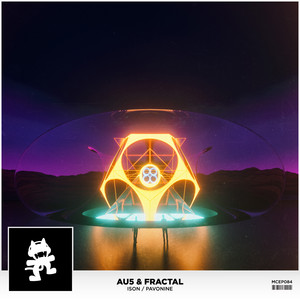## Ison / Pavonine

November 11, 2015

Play on spotify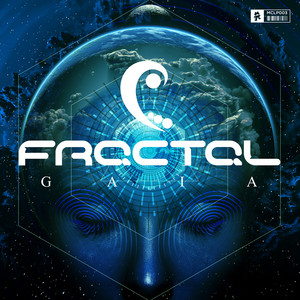## Gaia

October 7, 2015

Play on spotify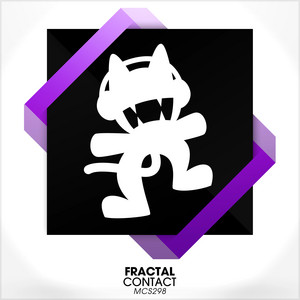## Contact

December 25, 2014

Play on spotify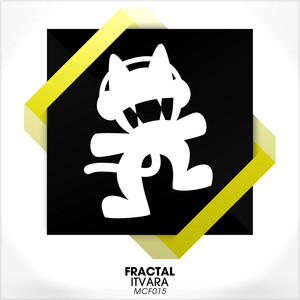## Itvara

April 16, 2014

Play on spotify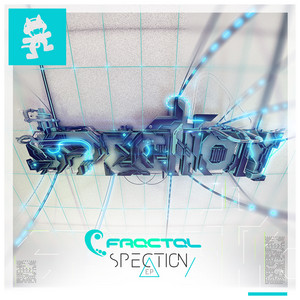## Spection

January 20, 2014

Play on spotify## Secret Weapon

December 1, 2013

Play on spotify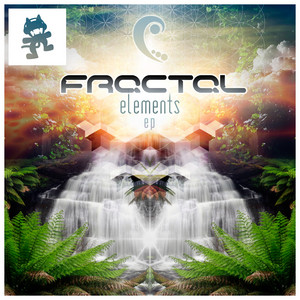## Elements

November 15, 2013

Play on spotify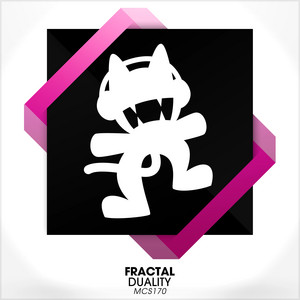## Duality

September 25, 2013

Play on spotify## Subvert

July 22, 2013

Play on spotify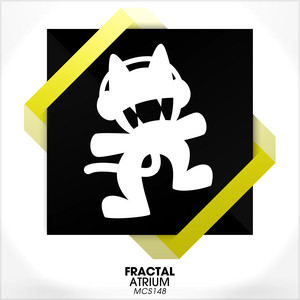## Atrium

July 8, 2013

Play on spotify